# What Is The Law Of Cosines? (5 Things You Need To Know)

The Law of Cosines is useful in geometry and trigonometry when we solve triangles (to find their side lengths and angle measures).

So, what is the Law of Cosines?  The Law of Cosines relates the sides & angles of a triangle, using the cosine function. If the triangle’s sides are a, b, & c, with side c across from angle C, then the Law of Cosines says that a2 + b2 – 2abcos(C) = c2. We can use this equation to solve for an unknown side or angle in a triangle.

Of course, there are some situations where we cannot use the Law of Cosines.  In those cases, the Law of Sines may be able to help us.

In this article, we’ll talk about the Law of Cosines, what it is, and where it comes from.  We’ll also go through some examples so you know how to use the Law of Cosines.

Let’s get started.

## What Is The Law Of Cosines?

The Law of Cosines relates the side lengths and angles of a triangle, using the cosine trigonometric function.

Assume that a triangle has side lengths a, b, and c, with angles A, B, and C.  Side a is opposite angle A, side b is opposite angle B, and side c is opposite angle C, as shown below:The Law of Cosines gives us an equation to relate the side lengths and angle measures of a triangle.

The Law of Cosines formula tells us that:

• a2 + b2 – 2abcos(C) = c2

We can also take the square root of both sides to solve for the side length c, which gives us the equation:

• c = √( a2 + b2 – 2abcos(C))

We can also solve for the side length a or b to get the equations:

• a = √(c2 – b2 + 2abcos(C))
• b = √(c2 – a2 + 2abcos(C))

### What Does The Law Of Cosines Find?

The Law of Sines allows us to relate four numbers: the three side lengths of a triangle and one of the angle measures.  The side-angle pair c and C are opposite (side c is across from angle C).

If we have any three of these four numbers, we can use the Law of Cosines to find the fourth one.  The Law of Cosines can be used in triangulation.

## When To Use The Law Of Cosines

The possible criteria for the Law of Cosines are as follows:

• 1. To find an unknown angle C in a triangle, given the lengths of all 3 sides.
• 2. To find an unknown side length in a triangle, given the lengths of the other 2 sides and one of the angles.

Note that in either case, we must know at least two side lengths, in addition to another side length or angle measure, to use the Law of Cosines.

The Law of Cosines works for all triangles: acute, right, and obtuse.  Of course, there is a special case of the Law of Cosines when an angle is 90 degrees (a right triangle)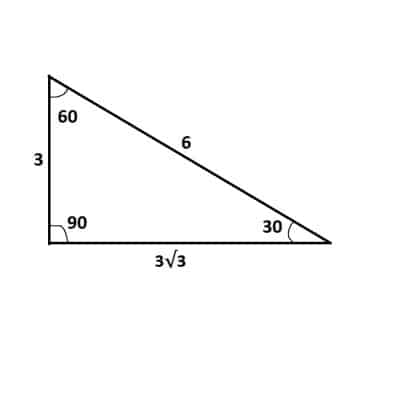In the special case of a right triangle (one with a 90 degree angle), the Law of Cosines simplifies to the Pythagorean Theorem, since cos(90) = 0.

### Law Of Cosines Special Case (Pythagorean Theorem)

When we have a right triangle, let the right angle (90 degrees) be angle C in the Law of Cosines.  Then we can plug in C = 90 and simplify to get:

• a2 + b2 – 2abcos(C) = c2
• a2 + b2 – 2abcos(90) = c2  [use C = 90 for a right triangle]
• a2 + b2 – 2ab*0 = c2  [since cos(*90) = 0]
• a2 + b2 – 0 = c2
• a2 + b2 = c2

This is the same as the Pythagorean Theorem!  So, the Pythagorean Theorem is a special case of the Law of Cosines in the case when C = 90 degrees (a right triangle).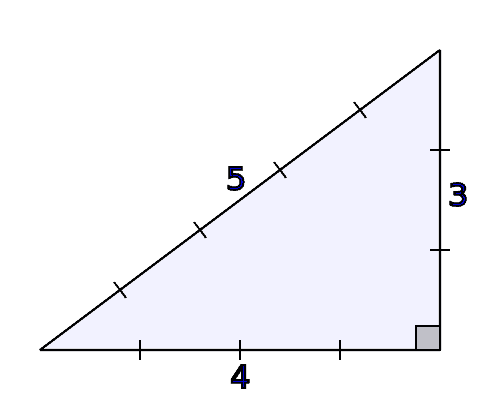A Pythagorean Triple (such as 3, 4, 5) represents the side lengths of a right triangle.

### How To Find An Angle Using The Law Of Cosines

To find an angle using the Law of Cosines, we must have:

• The three side lengths of the triangle.

Let’s look at some examples to see how it works in practice.

#### Example 1: How To Find An Angle Using The Law Of Cosines

Let’s say that we have the following triangle: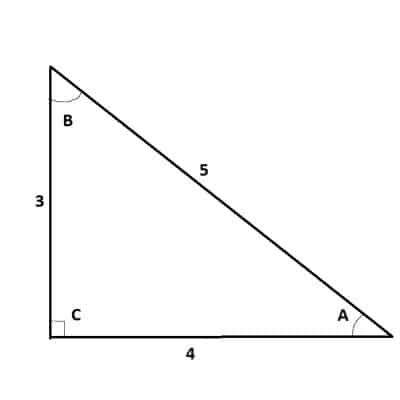We will use the Law of Cosines with a = 3, b = 4, and c = 5 to solve this triangle.

We will apply the Law of Cosines with a = 3, b = 4, and c = 5 to find angle C.  Using the Law of Sines equation with these values gives us:

• a2 + b2 – 2abcos(C) = c2
• 32 + 42 – 2(3)(4)cos(C) = 52
• 9 + 16 – 24cos(C) = 25
• 25 – 24cos(C) = 25
• –24cos(C) = 0
• cos(C) = 0
• C = 90

So, the measure of angle C is 90 degrees.  This means that the triangle is a right triangle (the side lengths are the Pythagorean Triple 3, 4, 5).

Since we have a right triangle, we can use a trigonometric identity to find the other angles.  If we take the sine of angle A (across from side a), we get:

• sin(A) = a/c
• sin(A) = 3/5
• A = sin-1(0.6)

So, angle A is approximately 36.9 degrees.  Since the angles in a triangle add up to 180, we can find B:

• A + B + C = 180
• 36.9 + B + 90 = 180
• 126.9 + B = 180
• B = 53.1

The fully solved triangle is given below:

#### Example 2: How To Find An Angle Using The Law Of Cosines

Let’s say that we have the following triangle: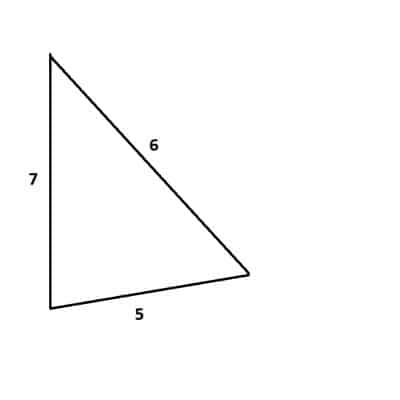We will use the Law of Cosines with a = 5, b = 6, and c = 7 to solve this triangle.

We will apply the Law of Cosines with a = 5, b = 6, and c = 7 to find angle C.  Using the Law of Sines equation with these values gives us:

• a2 + b2 – 2abcos(C) = c2
• 52 + 62 – 2(5)(6)cos(C) = 72
• 25 + 36 – 60cos(C) = 49
• 61 – 60cos(C) = 49
• – 60cos(C) = -12
• cos(C) = 1/5
• C = cos-1(0.2)

So, the measure of angle C is approximately 78.5 degrees.

We can now use the Law of Cosines (or the Law of Sines) to find the other angles.  Let’s use the Law of Sines to find angle A:

• a/sin(A) = c/sin(C)
• 5/sin(A) = 7/sin(78.5)
• 5sin(78.5) = 7sin(A)  [cross multiply to eliminate fractions]
• 5*0.98 = 7sin(A)
• 4.9 = 7sin(A)
• 0.7 = sin(A)
• sin-1(0.7) = A

So, angle A is approximately 44.4 degrees.  Since the angles in a triangle add up to 180, we can find B:

• A + B + C = 180
• 44.4 + B + 78.5 = 180
• 122.9 + B = 180
• B = 57.1

The fully solved triangle is given below:

### How To Find A Missing Side Using The Law Of Cosines

To find a missing side using the Law of Cosines, we must have:

• Any two side lengths and an angle from the triangle.

Let’s look at some examples to see how it works in practice.

#### Example 1: How To Find A Missing Side Using The Law Of Cosines (With Side-Angle Pair)

Let’s say that we have the following triangle: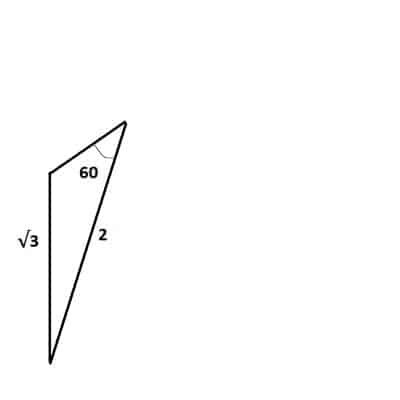We will use the Law of Cosines withb = 2, c = √3, and C = 60 to solve this triangle.

By labeling the sides and angles, we can see that we have the side-angle pair c and C, along with side b.  Here, we have b = 2, c = √3, and C = 60.

First, we’ll find side length a.  Using the Law of Cosines equation with these values gives us:

• a2 + b2 – 2abcos(C) = c2
• a2 + 22 – 2a(2)cos(60) = (√3)2
• a2 + 4 – 2a(2)(0.5) = 3  [since cos(60) = 0.5]
• a2 + 4 – 2a = 3
• a2 – 2a – 1 = 0
• (a – 1)2 = 0
• a – 1 = 0
• a = 1

So, the side length of a is 1.

Now we can use the Law of Sines (or the Law of Cosines) to find angles A and B.  Using the Law of Sines gives us:

• a/sin(A) = c/sin(C)
• 1/sin(A) = √3/sin(60)
• 1sin(60) = √3sin(A)  [cross multiply to eliminate fractions]
• 1/2 = √3sin(A)
• 1/2√3 = sin(A)
• sin-1(1/2√3) = A

So, angle A is approximately 16.8 degrees.  Since the angles in a triangle add up to 180, we can find B:

• A + B + C = 180
• 16.8 + B + 60 = 180
• 76.8 + B = 180
• B = 103.2

The fully solved triangle is given below:

#### Example 2: How To Find A Missing Side Using The Law Of Cosines (Without Side-Angle Pair)

Let’s say that we have the following triangle: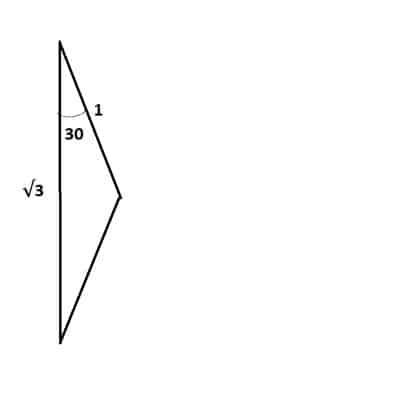We will use the Law of Cosines witha = 1, b = √3, and C = 30 to solve this triangle.

By labeling the sides and angles, we can see that we have a = 1, b = √3, and C = 30.

First, we’ll find side length c.  Using the Law of Cosines equation with these values gives us:

• a2 + b2 – 2abcos(C) = c2
• 12 + (√3)2 – 2(1)(√3)cos(30) = c2
• 1 + 3 – 2√3(√3/2) = c2  [since cos(30) = √3/2]
• 4 – 3 = c2
• 1 = c2
• 1 = c

So the side length c is 1.  This means that a and c have the same side length, which means that their opposite angles (A and C) are equal.  Since C = 30, then A = 30 also.

Since the angles in a triangle add up to 180, we can find B:

• A + B + C = 180
• 30 + B + 30 = 180
• 60 + B = 180
• B = 120

The fully solved triangle is given below:

## Why Does The Law Of Cosines Work? (How To Derive The Law Of Cosines: A Proof)

The Law of Cosines works because we can split any triangle into two right triangles and use the definition of the sine and cosine functions to find various lengths.

Let’s say we have the following triangle: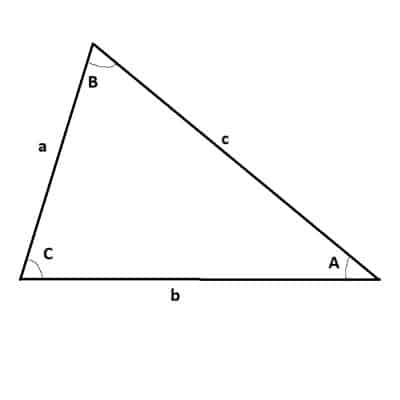Here is our triangle for the proof of the Law of Cosines, with all three sides and angles labeled.

First, we will draw a line segment from the vertex at angle B to the point P on side b, so that the line segment is perpendicular to side b: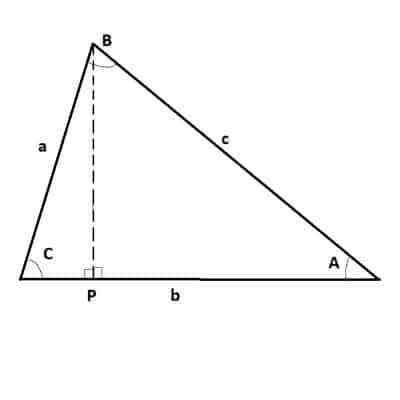The line segment from the vertex at angle B to the point P is a line segment that is horizontal to side b. This gives us two right triangles.

Next, we will label the perpendicular line segment H (the height of the triangle):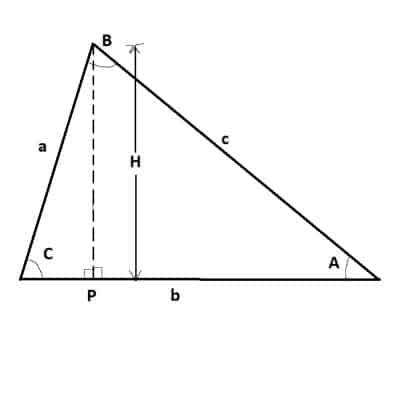The height of the perpendicular line segment is labeled as H. This is one of the side lengths of each right triangle.

Then, we will label the base of the left-most right triangle (adjacent to angle C) as “x”:

Then, we will take the sine of angle C (opposite divided by hypotenuse) in the left-most right triangle to get:

• sin(C) = H/a

Solving for H, we get:

• H = asin(C)

Then, we will take the cosine of angle C (adjacent divided by hypotenuse) in the left-most right triangle to get:

• cos(C) = x/a

Solving for x, we get:

• x = acos(C)

Now, we will label the base of the right-most right triangle (adjacent to angle A) as “b – x”: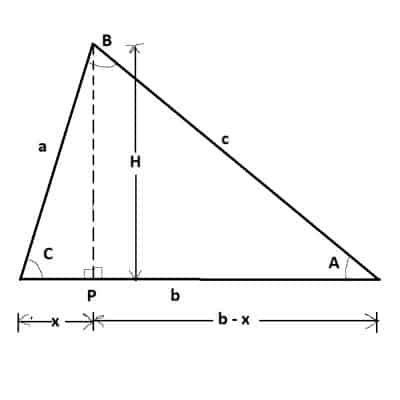The width of the base of the right-most right triangle is labeled as b – x.

Then, we use the Pythagorean Theorem for the three sides of the right-most right triangle to get:

• H2 + (b – x)2 = c2
• (asin(C))2 + (b2 – 2bx + x2) = c2  [since H = asin(C)]
• (asin(C))2 + b2 – 2b(acos(C) + (acos(C))2 = c2  [since x = acos(C)]
• a2sin2(C) + b2 – 2abcos(C) + a2cos2(C) = c2
• a2(sin2(C) + cos2(C)) + b2 – 2abcos(C) = c2
• a2(1) + b2 – 2abcos(C) = c2  [since sin2(C) + cos2(C) = 1 for any angle C]
• a2 + b2 – 2abcos(C) = c2

So, we get the Law of Cosines from the triangle.

## Conclusion

Now you know what the Law of Cosines is and what it is used for.  You also know how to solve a triangle using the Law of Cosines.

You can learn about the Triangle Inequality here.

You can learn about the output values of cosine here.